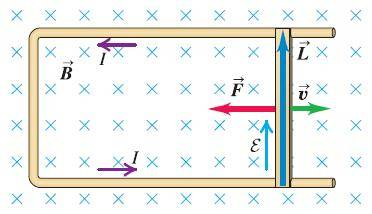# Can the Lorentz force explain the following phenomenon?

Coffee_The situation is probably well known by many of you. The setup is at rest realtive to the B-field while the rod is moving at a constant velocity. I have been thinking about the connection between the Lorentz force and Maxwell 3 and looking stuff up about it.

The first question is wheither only electrostatics and the lorentzforce can explain everything that will be happening in this phenomenon? So basically, the fact that this thing will act exactly in the same way as if instead of the rod there was a battery with a certain voltage that is equal to the EMF of the circuit.

In class I remember doing so, and even arriving at ##EMF=-\frac{d\phi}{dt}## again using only forces. This seems to give the exact same answer as Maxwells third law for this situation. After looking stuff up, it seems that the Lorentz force and Maxwell 3 are different things and still give the same result here. I'm just hoping that someone sees where my confusion lies because I can't even explain it decently it seems.

Gold Member
Of course the basics of the phenomenon is the effect of the Lorentz force on the electrons and so, inevitably, Lorentz force is one way of accounting for it. But the point is, the Lorentz force is given for the force the fields exert on a single charge and it needs some work for being used here. Maxwell's equations, on the other hand, are empirical laws coming from exactly such experiments and so are suited for such situations. You should see that Maxwell's equations are wrapping up all the behaviour of classical EM, but the Lorentz force equation is only the part that the fields affect charges.

Coffee_
Of course the basics of the phenomenon is the effect of the Lorentz force on the electrons and so, inevitably, Lorentz force is one way of accounting for it. But the point is, the Lorentz force is given for the force the fields exert on a single charge and it needs some work for being used here. Maxwell's equations, on the other hand, are empirical laws coming from exactly such experiments and so are suited for such situations. You should see that Maxwell's equations are wrapping up all the behaviour of classical EM, but the Lorentz force equation is only the part that the fields affect charges.

Then I'd like to ask where the following reasoning using Maxwell's equation goes wrong here:

##\nabla \times \vec{E} =-\frac{\partial \vec{B}}{\partial t}## , since in the frame of the setup or the U-shapend conductor (not the rod) at no place the ##\vec{B}## is changing it can be concluded that ##\nabla \times \vec{E}=0##. This would mean that in this frame Maxwell's third law is useless.

Edit: forgot curl sign

Gold Member
Then I'd like to ask where the following reasoning using Maxwell's equation goes wrong here:

##\nabla \vec{E} =\frac{\partial \vec{B}}{\partial t}## , since in the frame of the setup or the U-shapend conductor (not the rod) at no place the ##\vec{B}## is changing it can be concluded that ##\nabla \vec{E}=0##. This would mean that in this frame Maxwell's third law is useless.

Well, Maxwell's equations have differential and integral forms. Here it seems that the integral form of the Faraday's law should be considered which gives the right answer.
Actually I feel Maxwell's equations in differential form are more suited for applications in problems where we don't deal with circuits. In fact we got them from the integral forms to free them from surfaces and curves so that we can use them in free space and out of any wire.

Homework Helper
See here for a derivation

Coffee_
Well, Maxwell's equations have differential and integral forms. Here it seems that the integral form of the Faraday's law should be considered which gives the right answer.
Actually I feel Maxwell's equations in differential form are more suited for applications in problems where we don't deal with circuits. In fact we got them from the integral forms to free them from surfaces and curves so that we can use them in free space and out of any wire.

The integral form won't change anything it seems to me:

##\nabla \times \vec{E} =- \frac{\partial \vec{B}}{\partial t}##

##\iint\limits_\Sigma \nabla \times \vec{E} d\vec{S} = -\iint\limits_\Sigma \frac{\partial \vec{B}}{\partial t} d\vec{S} ##

The first can be transformed into a line integral according to stokes law in any case:

##\int\limits_S \vec{E} d\vec{s} = - \iint\limits_\Sigma \frac{\partial \vec{B}}{\partial t} d\vec{S} ##

However you can't pull the time derivative out of the integral because the integral operator changes in time in this case namely, the surface ##\Sigma## changes. This doesn't change anything of the fact that if ##\frac{\partial \vec{B}}{\partial t}=0## Maxwell 3 is useless.

Coffee_
The integral form won't change anything it seems to me:

##\nabla \times \vec{E} = -\frac{\partial \vec{B}}{\partial t}##

##\iint\limits_\Sigma \nabla \times \vec{E} d\vec{S} =- \iint\limits_\Sigma \frac{\partial \vec{B}}{\partial t} d\vec{S} ##

The first can be transformed into a line integral according to stokes law in any case:

##\int\limits_S \vec{E} d\vec{s} = - \iint\limits_\Sigma \frac{\partial \vec{B}}{\partial t} d\vec{S} ##

However you can't pull the time derivative out of the integral because the integral operator changes in time in this case namely, the surface ##\Sigma## changes. This doesn't change anything of the fact that if ##\frac{\partial \vec{B}}{\partial t}=0## Maxwell 3 is useless.

Coffee_
See here for a derivation

Thanks I checked it out. This one seems to assume Faraday's induction is true. I'd like to assume Maxwell and Lorentz force are true. What I'm wondering about is which one do I need to explain the phenomenon I talked about in my original post classically (that is not invoking special relativity which is closely related to what I'm asking I think). So anyway check my other answer, to me it seems that Maxwell 3 would be useless in this situation or do I make a reasoning mistake somewhere?